# Jacobian Method

Jacobi iterative method is considered as an iterative algorithm which is used for determining the solutions for the system of linear equations in numerical linear algebra, which is diagonally dominant. In this method, an approximate value is filled in for each diagonal element. Until it converges, the process is iterated. This algorithm was first called the Jacobi transformation process of matrix diagonalization.

Let the n system of linear equations be Ax = b.

Here,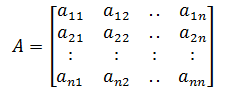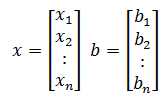Let us decompose matrix A into a diagonal component D and remainder R such that A = D + R.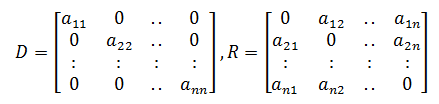Iteratively the solution will obtain using the below equation.

x(k+1) = D-1(b – Rx(k))

Here,

xk = kth iteration or approximation of x

x(k+1) = Next iteration of xk or (k+1)th iteration of x

The formula for the element-based method is given as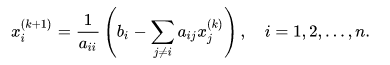## Jacobian Method Formula

Given an exact approximation x(k) = (x1(k), x2(k), x3(k), …, xn(k)) for x, the procedure of Jacobian’s method helps to use the first equation and the present values of x2(k), x3(k), …, xn(k) to calculate a new value x1(k+1). Likewise, to evaluate a new value xi(k) using the ith equation and the old values of the other variables. That is, given current values x(k) = (x1(k), x2(k), …, xn(k)), determine new values by solving for x(k+1) = (x1(k+1), x2(k+1), …, xn(k+1)) in the below expression of linear equations.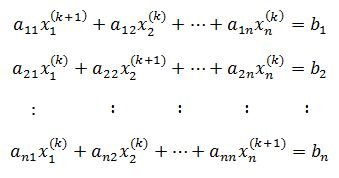The above system of equations can also be written as below.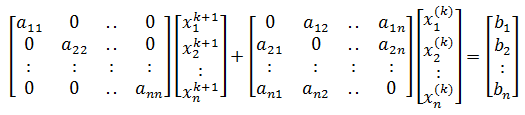From the above expression it is clear that, the subscript i indicates that xi(k) is the ith element of vector x(k) = (x1(k), x2(k), …, xi(k), …, xn(k) ), and superscript k corresponds to the particular iteration (not the kth power of xi ).

Assume that D, U, and L represent the diagonal, strict upper triangular and strict lower triangular and parts of matrix A, respectively, then the Jacobian’s method can be described in matrix-vector notation as given below.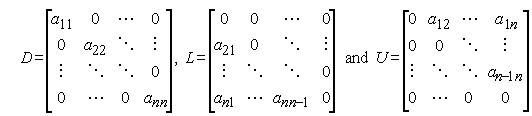This can be simplified as shown below.

Dx(k+1) + (L + U)x(k) = b

x(k+1) = D-1[(-L-U)x(k) + b]

## Properties of Jacobian Method

Adding the applications of Jacobian matrix in different areas, this method holds some important properties. The simplicity of this method is considered in both the aspects of good and bad. This method can be stated as good since it is the first iterative method and easy to understand. However, the method is also considered bad since it is not typically used in practice. Though there are cons, still it is a good starting point for those who are willing to learn more useful but more complicated iterative methods.

### Jacobian Method Example

A system of linear equation of the form Ax = b with an initial estimate x(0) is given below.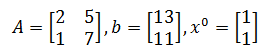Solve the above using the Jacobian method.

Solution:

Given,We know that x(k+1) = D-1(b – Rx(k)) is used to estimate x.

Let us rewrite the above expression in a more convenient form, i.e. D-1(b – Rx(k)) = Tx(k) + C

Here,

T = -D-1R

C = D-1b

R = L + U

Let us split the matrix A as diagonal matrix and remainder.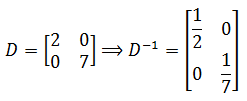Upper and lower parts of reminder of A: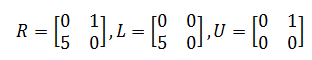Here,

R = Remainder matrix

L = Lower part of R

U = Upper part of R

T = -D-1(L + U) = D-1[-L + (-U)]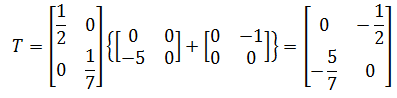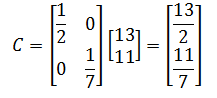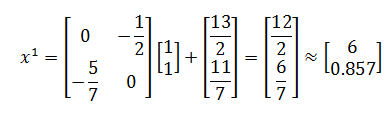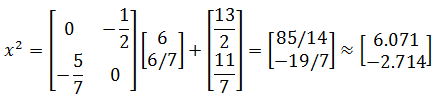Repeat the above process until it converges, i.e. until the value of ||Axn – b|| is small.